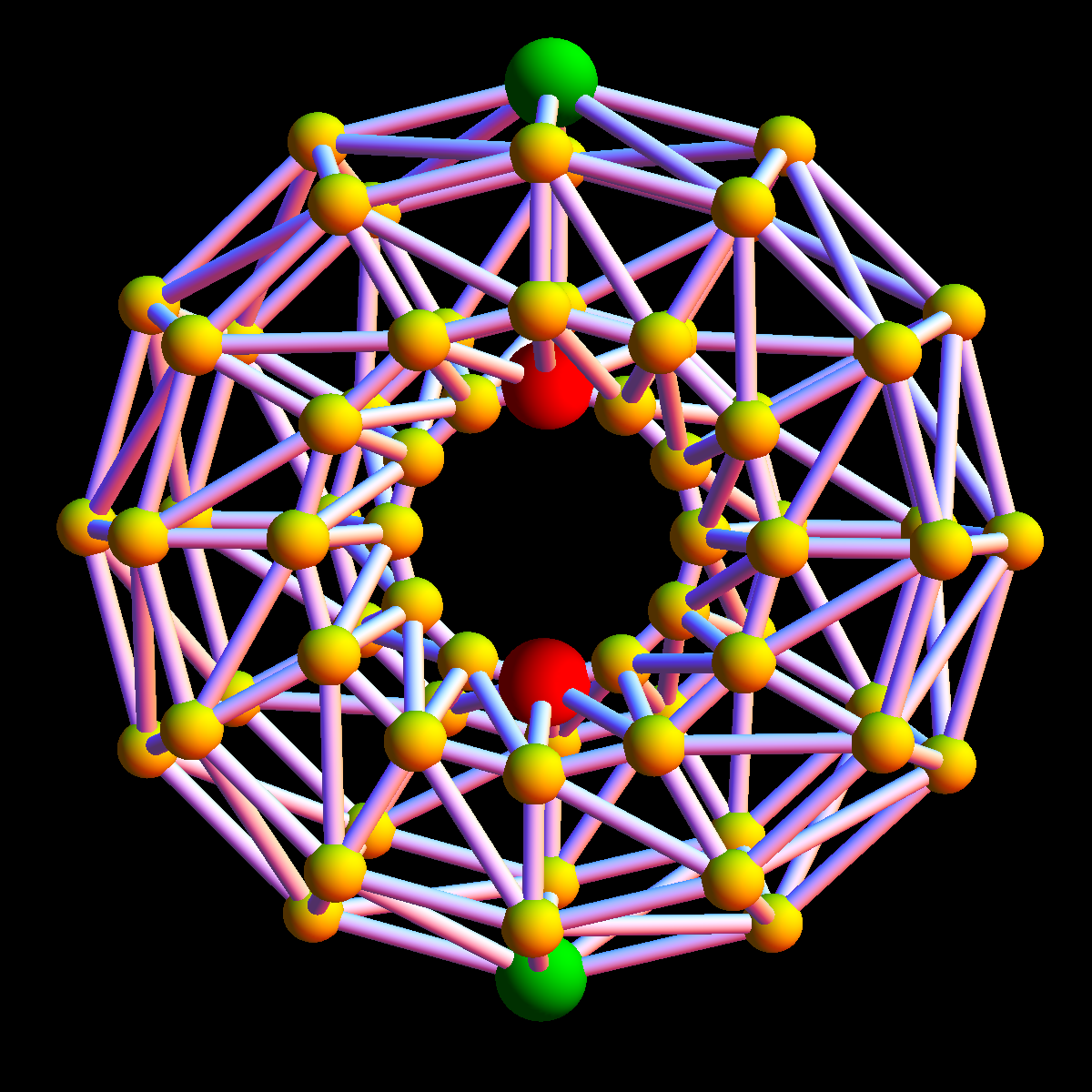# Exhibit: Poincare Hopf

 Theorem: (Details) Let (V,E) be an arbitrary simple graph and f an injective function on V. The sum of the integer indices i(v) of f on critical points of f is the Euler characteristic X(G). Here i(v) = 1-X(S-(v)), where S-(v) is the subset of the neighbors of v, where f(w) is smaller than f(v). For example, if v is a minimum, then X- is empty and i(v)=1. The next picture shows an illustration in a situation close to the continuum, where a graph is a discrete torus embedded in space and slightly tilted so that height is injective. Then there are two critical points with index 1 and 2 critical points with index -1: For the minimum at the bottom, the set S-(v) is empty. Its Euler characteristic is 0 and the index is i(v) = 1-0 = 1. For the maximum v on the top, the set set S- is a cyclic graph of order 6. Its Euler characteristic is 0. Therefore i(v) = 1-0 = 1. For the lower red vertex, the set S- consists of two linear "interval" graphs ((A,B,C),A->B,B->C) of order 3 and size 2. The Euler characteristic of each "interval" is 1, the Euler characteristic of S- is 2. The index is 1-2=-1. For the upper red vertex, the set S- consists of two isolated points (A,()) of order 1 and size 0. The Euler characteristic of each "point" is 1, the Euler characteristic of S- is 2. The index is 1-2=-1. If we add up the sum of the indices over V, we get the Euler characteristic, which is zero in this case. Note however that the above theorem is very general. It works for any simple graph. The graph does not have to have to be a discretisation of a manifold.This page posted Jan 8, 2012